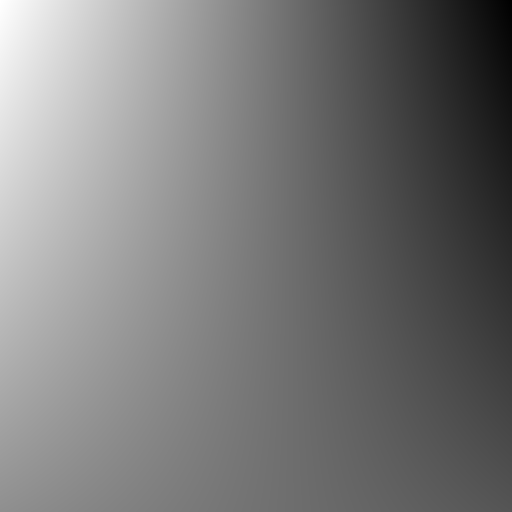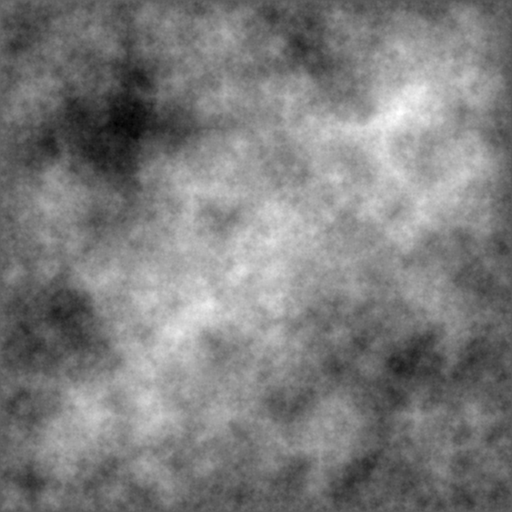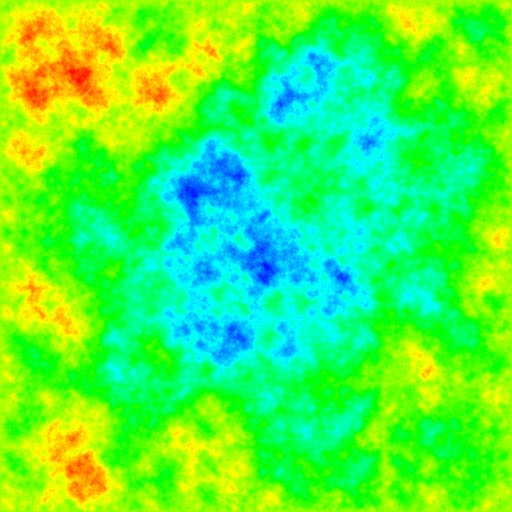## Monday, 19 January 2015

### The Diamond Square Algorithm

Ever wondered how to generate a landscape?  I've been fascinated by these since the days of Vista Pro on my trusty Amiga.

The diamond-square algorithm is a method for generating heightmaps.  It's a great algorithm because it's amazingly simple and produces something very visual (similar to the emergent behaviour exhibited by the flocking algorithm.  My kind of algorithm!  In this post, I'll try to explain the implementation using Haskell and generate some pretty pictures.

As the paper [PDF] states, previous modelling techniques for graphics were based on the idea that you can simply describe a landscape as some set of deterministic functions.  Bezier and B-spline patches used higher-order polynomials to describe objects and this approach was good for rendering artificial objects.  Natural objects, such as terrain, don't have regular patterns so an approach likes splines doesn't work.

This algorithm was innovative because it used a stochastic approach.  Given some simple rules (and some randomness!) the algorithm generates a "natural" looking landscape.  The paper describes models for 1D, 2D and 3D surfaces.  We'll just use the simplest possible example, rendering a height map.

We start with a square with each corner given a randomly assigned height.  If the area of this square is 1, then we’re done.  Easy.  We’ll call the corners, TL, TR, BL and BR (representing top left, top right, bottom left and bottom right).If the square is too big, then we recursively divide it into smaller squares.We assign each new square a height based on the average of the points surrounding it.  Note there’s nothing stochastic about this approach yet, it’s purely deterministic.

We can model this with Haskell pretty clearly.  We start off by defining a simple type to represent a Square.

type Point = (Int,Int)
data Square = Square
{
position :: Point
, size    :: Int
, tl      :: Double -- Height of top left
, tr      :: Double -- Height of top right
, bl      :: Double -- Height of bottom left
, br      :: Double -- Height of bottom right
} deriving (Show,Eq)

Now all we have to do write a little function to divide things into four.  Firstly let’s capture the pattern that dividing stops when the size of the square is one.

isUnit :: Square -> Bool
isUnit sq = size sq == 1

allSubSquares :: (Square -> [Square]) -> Square -> [Square]
allSubSquares f sq
| isUnit sq = [sq]
| otherwise = concatMap (allSubSquares f) (f sq)

The allSubSquares function now simply repeatedly called our splitting function until things are reduced to the tiniest possible size.

What does our split function look like?  Well, all it has to do is calculate the new squares as the picture defines above.  It looks a little like this:

divide :: Double -> Square -> [Square]
divide eps parent = [
sq                    { tr = at, br = ah, bl = al } -- top left unchanged
, (move sq (half,0))    { tl = at, bl = ah, br = ar } -- top right unchanged
, (move sq (0,half))    { tr = ah, br = ab, tl = al } -- bottom left unchanged
, (move sq (half,half)) { tl = ah, bl = ab, tr = ar } -- bottom right unchanged
]
where
half = size parent `div` 2
sq = parent { size = half }
at = averageTopHeight parent
ah = averageHeight eps parent -- height of middle
ab = averageBottomHeight parent
ar = averageRightHeight parent
al = averageLeftHeight parent

OK, this isn’t very exciting (and I’ve left out the boilerplate).  But we have something now, it’s deterministic, but it creates cool results.Woo. I used JuicyPixels to render the image.  I really wish I’d found this library a long time ago, it’s fabulously simple to use and all I needed to do was use the sexy generateImage function.

So how do we actually generate something that looks vaguely natural?

The answer is randomness.  Tightly controlled.  Let’s look at our original square divider and make one really small change.I’ll save you the trouble of finding it, it’s that pesky “e” we’ve added to the middle.  What is e?

Well, it’s the stochastic approach.  It’s a random number that’s assigned to displace the midpoint.  When the square is big, the displacement is big.  When the square is small, the displacement is small.  In fact we simply define e as a random number [-0.5, 0.5] scaled by the size of the square.

What happens when we add this displacement is kind of cool.  We now get a random surface that smooths itself out and almost looks natural.I think this is pretty neat.  It’s a smooth landscape that could easily look natural.  We can do even better by giving a bit of color.  I’ve done this using a simple color map as described on Stackoverflow.

Using a map generated from similar parameters, we get a much prettier colour.  If you squint a bit, imagine something it could be a natural scene right?All the code for this is available on my GitHub profile, the diamond-square project.  Some fun extensions to this would be to create some animations, or actually render it in 3D with OpenGL.